Related Articles

# Basic Conversion of Logic Gates

• Last Updated : 25 Feb, 2021

In the digital system, logic gates are the basic building blocks.  In these logic gates, we can find the gates having more than one input, but will have only one output. The connection between the input and the output of a gate is based on some logic. Based on this logic, different gates are developed like OR gate, AND gate, NOT gate, and more.   The gates that are developed have been divided into categories like Basic Gates, Universal Gates, and more.

Here we have used 2 inputs, and the combination of the inputs that we will have is 4. So we can have more than one or two inputs, and the combination of the input will be based on 2n. ‘n’ states the number of inputs of the logic gate.
For example:- if the number of input is 3, then the combination of the input will be 23 = 8.

Some Basic Conversion of Gates: –

AND to NAND: –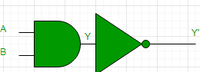The algebraic expression of the AND to NAND Gate conversion is Y=A’+B‘.

OR to NOR: –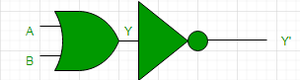The algebraic expression of the NOR gate is Y=A’.B’.

NAND to AND: –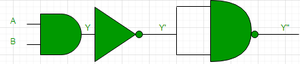The algebraic expression of the NAND to AND gate conversion is Y”=A.B.

After complement, we get the expression of the And gate.

NAND to OR: –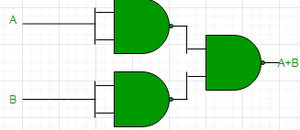The algebraic expression of the NAND to OR gate is- Y=A+B.

NOR to AND: –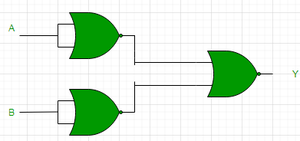The algebraic expression of the NOR to AND gate is Y=A.B.

NOR to NOT: –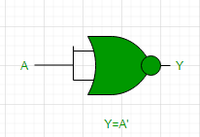The algebraic expression of the NOR to NOT gate conversion will be the same as the NOT gate. So the algebraic conversion of the NOT gate is:- Y=A’

NAND to NOT: –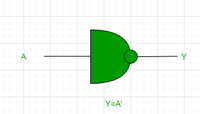The algebraic expression of the NAND to NOT gate conversion will be the same as the NOT gate. So the algebraic conversion of the NOT gate is:- Y=A’

NOR to OR: –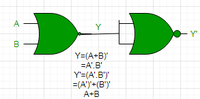The algebraic expression of the NOR to OR gate is: A+B

NOR to NAND: –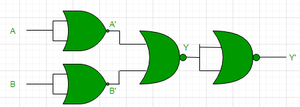The algebraic expression of the NOR to NAND gate is A’+B’

NOR to XOR: –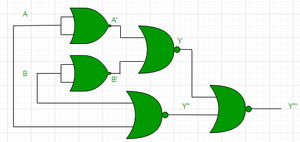The algebraic expression of the NOR to XOR gate is (A+(A+B)’)’+(B+(A+B)’)’

NOR to X-NOR: –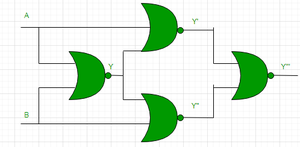The algebraic expression of the NOR to XNOR gate is- (A+B’). (A’+B)

Attention reader! Don’t stop learning now.  Practice GATE exam well before the actual exam with the subject-wise and overall quizzes available in GATE Test Series Course.

Learn all GATE CS concepts with Free Live Classes on our youtube channel.

My Personal Notes arrow_drop_up